# Control of a Multi-Input Multi-Output Nonlinear Plant

This example shows how to design a model predictive controller for a multi-input multi-output nonlinear plant. The plant has 3 manipulated variables and 2 measured outputs.

### Linearize Nonlinear Plant

```if ~mpcchecktoolboxinstalled('simulink') disp('Simulink(R) is required to run this example.') return end if ~mpcchecktoolboxinstalled('slcontrol') disp('Simulink Control Design(R) is required to run this example.') return end ```

The nonlinear plant is implemented in Simulink model `mpc_nonlinmodel` and linearized at the default operating condition using the `linearize` command from Simulink Control Design.

```plant = linearize('mpc_nonlinmodel'); ```

Assign names to I/O variables.

```plant.InputName = {'Mass Flow';'Heat Flow';'Pressure'}; plant.OutputName = {'Temperature';'Level'}; plant.InputUnit = {'kg/s' 'J/s' 'Pa'}; plant.OutputUnit = {'K' 'm'}; ```

### Design MPC Controller

Create the controller object with sampling period, prediction and control horizons:

```Ts = 0.2; p = 5; m = 2; mpcobj = mpc(plant,Ts,p,m); ```
```-->The "Weights.ManipulatedVariables" property of "mpc" object is empty. Assuming default 0.00000. -->The "Weights.ManipulatedVariablesRate" property of "mpc" object is empty. Assuming default 0.10000. -->The "Weights.OutputVariables" property of "mpc" object is empty. Assuming default 1.00000. ```

Specify MV constraints.

```mpcobj.MV = struct('Min',{-3;-2;-2},'Max',{3;2;2},'RateMin',{-1000;-1000;-1000}); ```

Define weights on manipulated and controlled variables.

```mpcobj.Weights = struct('MV',[0 0 0],'MVRate',[.1 .1 .1],'OV',[1 1]); ```

Run simulation.

```mdl1 = 'mpc_nonlinear'; open_system(mdl1) sim(mdl1) ```
```-->Converting model to discrete time. -->Assuming output disturbance added to measured output channel #1 is integrated white noise. -->Assuming output disturbance added to measured output channel #2 is integrated white noise. -->The "Model.Noise" property of the "mpc" object is empty. Assuming white noise on each measured output channel. ```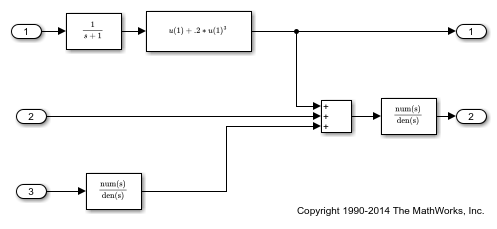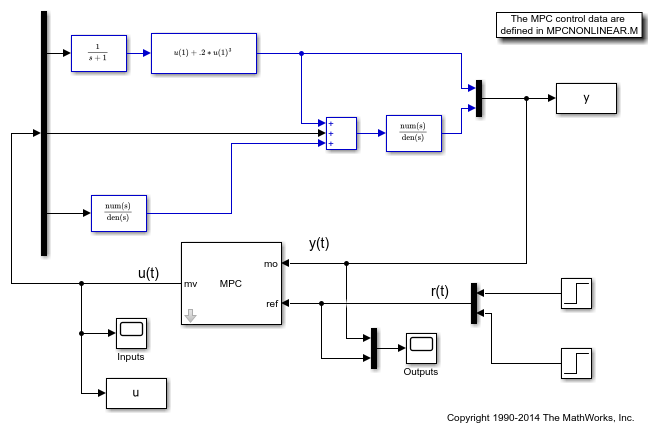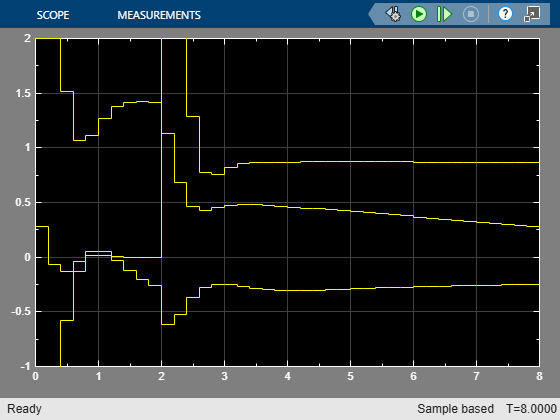### Modify MPC Design to Track Ramp Signals

In order to track a ramp, a triple integrator is defined as an output disturbance model on both outputs.

```outdistmodel = tf({1 0;0 1},{[1 0 0 0],1;1,[1 0 0 0]}); setoutdist(mpcobj,'model',outdistmodel); ```

Run simulation.

```mdl2 = 'mpc_nonlinear_setoutdist'; open_system(mdl2) sim(mdl2) ```
```-->Converting model to discrete time. -->The "Model.Noise" property of the "mpc" object is empty. Assuming white noise on each measured output channel. ```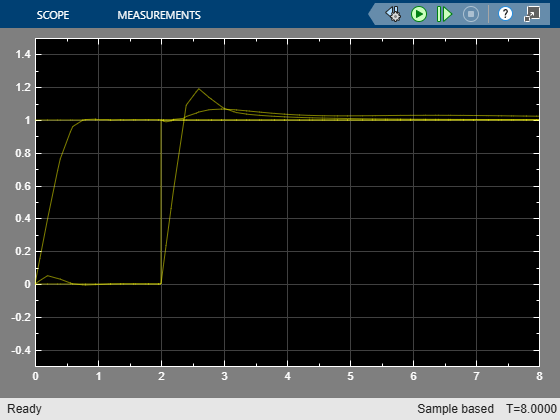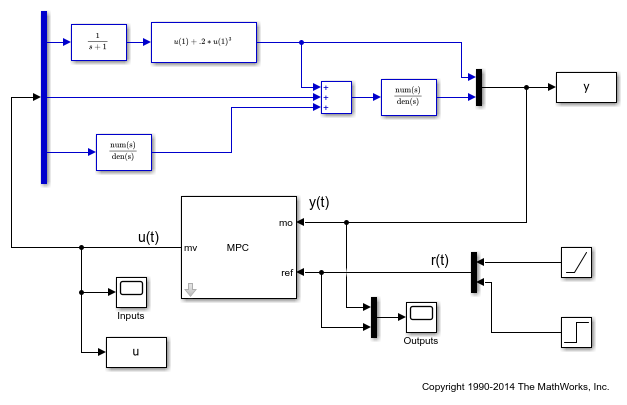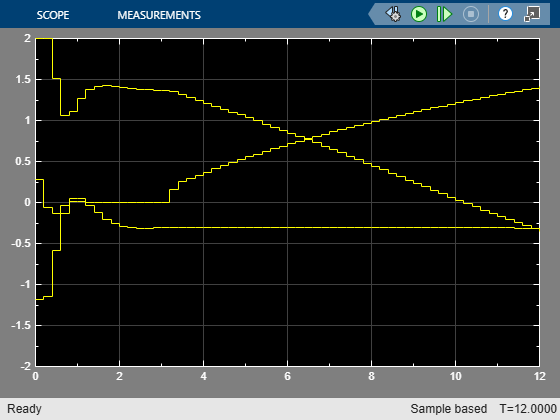### Simulate without Constraints

When the constraints are not active, the MPC controller behaves like a linear controller.

```mpcobj.MV = []; ```

Reset output disturbance model to default

```setoutdist(mpcobj,'integrators'); ```

The input to the linear controller LTI is the vector [ym;r], where ym is the vector of measured outputs, and r is the vector of output references.

```LTI = ss(mpcobj,'r'); ```
```-->Converting model to discrete time. -->Assuming output disturbance added to measured output channel #1 is integrated white noise. -->Assuming output disturbance added to measured output channel #2 is integrated white noise. -->The "Model.Noise" property of the "mpc" object is empty. Assuming white noise on each measured output channel. ```

Run simulation.

```refs = [1;1]; % output references are step signals mdl3 = 'mpc_nonlinear_ss'; open_system(mdl3) sim(mdl3) ```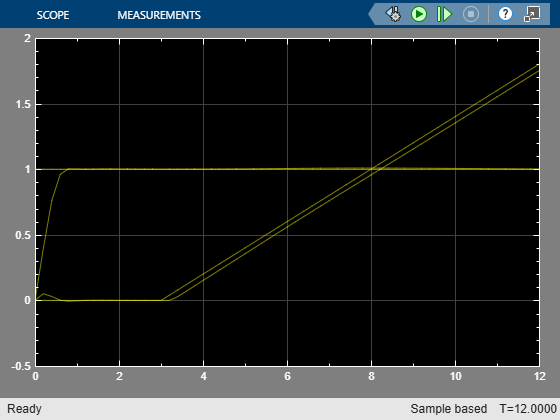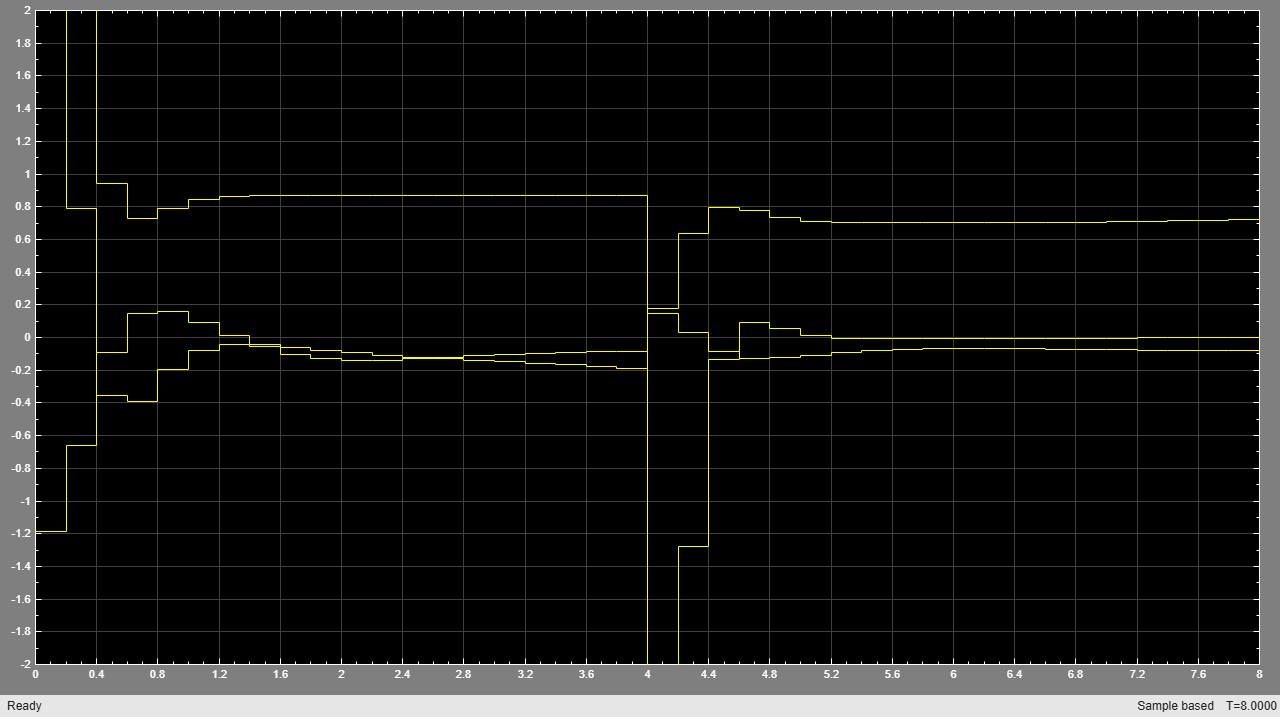### Compare Simulation Results

```fprintf('Compare output trajectories: ||ympc-ylin|| = %g\n',norm(ympc-ylin)); disp('The MPC controller and the linear controller produce the same closed-loop trajectories.'); ```
```Compare output trajectories: ||ympc-ylin|| = 1.73191e-14 The MPC controller and the linear controller produce the same closed-loop trajectories. ```
```bdclose(mdl1) bdclose(mdl2) bdclose(mdl3) ```